# College Physics

Physics & Astronomy

## Quiz 11 :Optical InstrumentsLooking for Introductory Physics Homework Help?

## Quiz 11 :Optical Instruments

Showing 1 - 20 of 77A lens is used to examine an object across a room. Is the lens probably being used as a simple magnifier Explain in terms of focal length, the image, and magnification.
Free
Essay

The magnification of a magnifier depends upon the near point of the person and the focal length of the lens. A magnifier forms an enlarged, upright and virtual image of an object at or inside the focal point of the lens. A lens with short focal length can be used to get high magnifications and so an enlarged virtual image.
Here the person will be observing the object across a room with the object being at large distances and the image being observed at a small distance from the lens. So the magnitude of the magnification of the lens would be less than one. Hence, the person will not be using the lens as a magnifier.A lens has a focal length of 28 cm and a diameter of 4.0 cm. What is the f -number of the lens
Free
Essay

Use the relation between focal length, diameter, and f - number of the lens to solve for the
f - number of the lens.
The expression which relates focal length, diameter, and f - number of the lens is,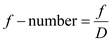Here, f is the focal length of the lens, and D  is the diameter of the lens.
Substitute 28 cm for f , and 4.0 cm for D in the equationto solve for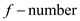.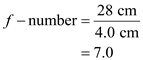Therefore, the f - number of the lens is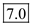.A laser beam is incident at a shallow angle on a horizontal machinist's ruler that has a finely calibrated scale. The engraved rulings on the scale give rise to a diffraction pattern on a vertical screen. Discuss how you can use this technique to obtain a measure of the wavelength of the laser light.
Free
Essay

A laser beam is incident at a shallow angle on a horizontal machinist's ruler that has a finely calibrated scale and this engraved rulings on the scale give rise to a diffraction pattern on a vertical screen as shown in the below figure.
Figure (a) shows the diffraction pattern produced by a laser light incident on scale.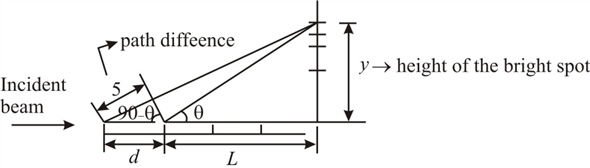Figure (a)
Light from the laser beam is nearly parallel to the horizontal ruler will scatter from ruler marks, which are separated by a distance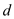apart to produce a diffraction pattern on a vertical wall at a distance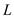away.
The condition for constructive interference is,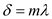Here,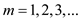The figure (a) includes two triangles. Redraw the first triangle as shown below.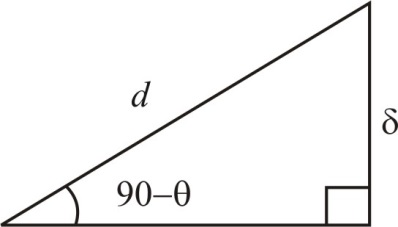From above figure, the sin angle is,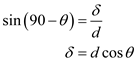From the second triangle, the tan angle is,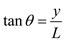For a small angle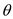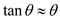.
Then above equation changes as,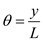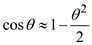Substitute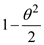for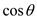and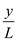forin equation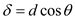.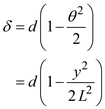Substitute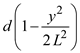for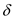in equationand solve for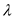.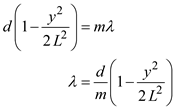Since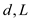and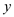are measurable, the wavelength of the laser beam can be calculated by measuring the height of the bright spots as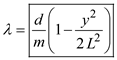.A certain camera has f -numbers that range from 1.2 to 22. If the focal length of the lens is 55 mm, what is the range of aperture diameters for the camera
EssayThe optic nerve and the brain invert the image formed on the retina. Why don't we see everything upside down
EssayA photographic image of a building is 0.092 0 m high. The image was made with a lens with a focal length of 52.0 mm. If the lens was 100 m from the building when the photograph was made, determine the height of the building.
EssaySuppose you are observing the interference pattern formed by a Michelson interferometer in a laboratory and a joking colleague holds a lit match in the light path of one arm of the interferometer. Will this match have an effect on the interference pattern
EssayThe image area of a typical 35 mm slide is 23.5 mm by 35.0 mm. If a camera's lens has a focal length of 55.0 mm and forms an image of the constellation Orion, which is 20° across, will the full image fit on a 35-mm slide
EssayIf you want to examine the fine detail of an object with a magnifying glass with a power of 120.0 diopters, where should the object be placed so as to observe a magnified image of the object
EssayA camera is being used with a correct exposure at f /4 and a shutter speed of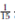s. In addition to the f -numbers listed in Section 25.1, this camera has f -numbers f /1, f /1.4, and f /2. To photograph a rapidly moving subject, the shutter speed is changed to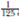s. Find the new f -number setting needed on this camera to maintain satisfactory exposure.
EssayCompare and contrast the eye and a camera. What parts of the camera correspond to the iris, the retina, and the cornea of the eye
Essay(a) Use conceptual arguments to show that the intensity of light (energy per unit area per unit time) reaching the film in a camera is proportional to the square of the reciprocal of the f -number as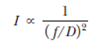(b) The correct exposure time for a camera set to f /1.8 is (1/500) s. Calculate the correct exposure time if the f -number is changed to f /4 under the same lighting conditions. Note: " f /4," on a camera, means "an f - number of 4."
EssayIf you want to use a converging lens to set fire to a piece of paper, why should the light source be farther from the lens than its focal point
EssayA certain type of film requires an exposure time of 0.010 s with an f /11 lens setting. Another type of film requires twice the light energy to produce the same level of exposure. What f -number does the second type of film need with the 0.010-s exposure time
EssayLarge telescopes are usually reflecting rather than refracting. List some reasons for this choice.
EssayA certain camera lens has a focal length of 175 mm. Its position can be adjusted to produce images when the lens is between 180 mm and 210 mm from the plane of the film. Over what range of object distances is the lens useful
EssayExplain why it is theoretically impossible to see an object as small as an atom regardless of the quality of the light microscope being used.
EssayThe near point of a person's eye is 60.0 cm. To see objects clearly at a distance of 25.0 cm, what should be the (a) focal length and (b) power of the appropriate corrective lens (Neglect the distance from the lens to the eye.)
Essay